Courses

# Vehicle Arrival Models: Headway Civil Engineering (CE) Notes | EduRev

## Civil Engineering (CE) : Vehicle Arrival Models: Headway Civil Engineering (CE) Notes | EduRev

The document Vehicle Arrival Models: Headway Civil Engineering (CE) Notes | EduRev is a part of the Civil Engineering (CE) Course Transportation Engineering.
All you need of Civil Engineering (CE) at this link: Civil Engineering (CE)

Introduction

Modelling arrival of vehicle at section of road is an important step in traffic flow modelling. It has important application in traffic flow simulation where vehicles are to be generated how vehicles arrive at a section. The vehicle arrival is obviously a random process. This is evident if one observe how vehicles are arriving at a cross section. Some times several vehicles come together, while at other times, they come sparsely. Hence, vehicle arrival at a section need to be characterized statistically. Vehicle arrivals can be modelled in two inter-related ways; namely modelling what is the time interval between the successive arrival of vehicles or modelling how many vehicle arrive in a given interval of time. In the former approach, the random variables the time denoting interval between successive arrival of vehicle can be any positive real values and hence some suitable continuous distribution can be used to model the vehicle arrival. In the later approach, the random variables represent the number of vehicles arrived in a given interval of time and hence takes some integer values. Here in this approach, a discrete distribution can be used to model the process. This chapter presents how some continuous distributions can be used to model the vehicle arrival process.

An important parameter to characterize the traffic is to model the inter-arrival time of vehicle at a section on the road. The inter-arrival time or the time headway is not constant due to the stochastic nature of vehicle arrival. A common way of modeling to treat the inter-arrival time or the time headway as a random variable and use some mathematical distributions to model them. The behavior of vehicle arrival is different at different flow condition. It may be possible that different distributions may work better at different flow conditions. Suppose the vehicle arrive at a point at time t1, t2, . . . . Then the time difference between two consecutive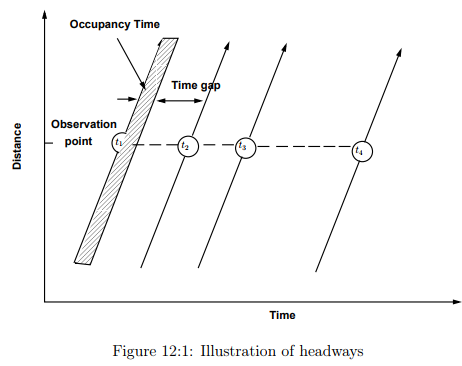arrivals is defined as headway. This is shown as a time-distance diagram in figure 12:1. In fact the headway consist of two components, the occupancy time which is the duration required for the vehicle to pass the observation point and the time gap between the rear of the lead vehicle and front of the following vehicle. Hence, the headways h1 = t2 − t1, h2 = t3 − t2, . . . It may be noted that the headways h1, h2, . . . will not be constant, but follows some random distribution. Further, under various traffic states, different distribution may best explain the arrival pattern. A brief discussion of the various traffic states and suitable distributions are discussed next.

Classification of traffic state

Generally, traffic state can be divided into three; namely low, medium and high flow conditions. Salient features of each of the flow state is presented below after a brief discussion of the probability distribution.

1. Low volume flow

(a) Headway follow a random process as there is no interaction between the arrival of two vehicles.

(b) The arrival of one vehicle is independent of the arrival of other vehicle.

(c) The minimum headway is governed by the safety criteria.

(d) A negative exponential distribution can be used to model such flow.

2. High volume flow

(a) The flow is very high and is near to the capacity.

(b) There is very high interaction between the vehicle.

(c) This is characterized by near constant headway.

(d) The mean and variance of the headway is very low.

(e) A normal distribution can used to model such flow.

3. Intermediate flow

(a) Some vehicle travel independently and some vehicle has interaction with other vehicles.

(b) More difficult to analyze, however, has more application in the field.

(c) Pearson Type III Distribution can be used which is a very general case of negative exponential distribution.

Negative exponential distribution

The low flow traffic can be modeled using the negative exponential distribution. First, some basics of negative exponential distribution is presented. The probability density function f(t) of any distribution has the following two important properties: First,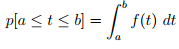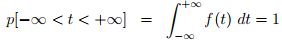(12.1)

where t is the random variable. This means that the total probability defined by the probability density function is one. Second:(12.2)

This gives an expression for the probability that the random variable t takes a value with in an interval, which is essentially the area under the probability density function curve. The probability density function of negative exponential distribution is given as: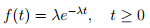(12.3)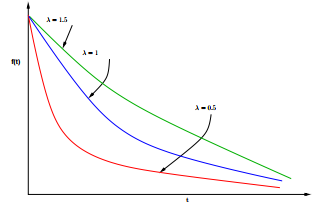Figure 12:2: Shape of the Negative exponential distribution for various values of λ

where λ is a parameter that determines the shape of the distribution often called as the shape parameter. The shape of the negative exponential distribution for various values of λ (0.5, 1, 1.5) is shown in figure 12:2. The probability that the random variable t is greater than or equal to zero can be derived as follow,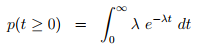(12.4)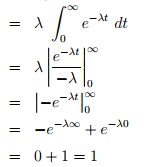The probability that the random variable t is greater than a specific value h is given as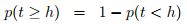(12.5)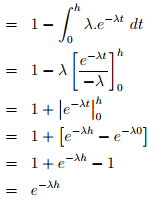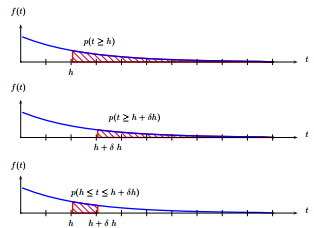Figure 12:3: Evaluation of negative exponential distribution for an interval

Unlike many other distributions, one of the key advantages of the negative exponential distribution is the existence of a closed form solution to the probability density function as seen above. The probability that the random variable t lies between an interval is given as: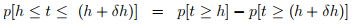(12.6)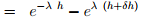This is illustrated in figure 12:3. The negative exponential distribution is closely related to the Poisson distribution which is a discrete distribution. The probability density function of Poisson distribution is given as: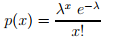(12.7)

where, p(x) is the probability of x events (vehicle arrivals) in some time interval (t), and λ is the expected (mean) arrival rate in that interval. If the mean flow rate is q vehicles per hour, then λ = q 3600 vehicles per second. Now, the probability that zero vehicle arrive in an interval t, denoted as p(0), will be same as the probability that the headway (inter arrival time) greater than or equal to t. Therefore,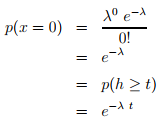Here, λ is defined as average number of vehicles arriving in time t. If the flow rate is q vehicles per hour, then,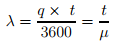(12.8)

Since mean flow rate is inverse of mean headway, an alternate way of representing the probability density function of negative exponential distribution is given as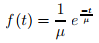(12.9)

where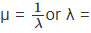1/µ. Here, µ is the mean headway in seconds which is again the inverse of flow rate. Using equation 12.6 and equation 12.5 the probability that headway between any interval and flow rate can be computed. The next example illustrates how a negative exponential distribution can be fitted to an observed headway frequency distribution.

Numerical Example

An observation from 2434 samples is given table below. Mean headway and the standard deviation observed is 3.5 and 2.6 seconds respectively. Fit a negative exponential distribution.

 h h + dh P°i 0.0 1.0 0.012 1.0 2.0 0.178 2.0 3.0 0.316 3.0 4.0 0.218 4.0 5.0 0.108 5.0 6.0 0.055 6.0 7.0 0.033 7.0 8.0 0.022 8.0 9.0 0.013 0.0 > 0.045 Total 1.00

Solution: The solution is shown in Table 12:2. The headway range and the observed probability (or proportion) is given in column (2), (3) and (4). The observed frequency for the first

interval (0 to 1) can be computed as the product of observed frequency pi and the number of observation (N). That is,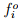= pi × N = 0.012 × 2434 = 29.21 and is shown in column (5). The probability that the headway greater than t = 0 is computed as p(t ≥ 0) = e −0 = 1 (refer equation 12.5) and is given in column (6). These steps are repeated for the second interval, that is= 0.178 × 2434 = 433.25, and p(t ≥ 1) = e −1 = 0.751. Now, the probability of headway lies between 0 and 1 for the first interval is given by the probability that headway greater than zero from the first interval minus probability that headway greater than one from second interval. That is pi(0 ≤ t ≤ 1) = pi(t > 0) − pi(t > 1) = 1.00 − 0.751 = 0.249 and is given in column (7). Now the computed frequency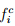is pi × N = 0.249 × 2434 = 604.904 and is given in column (8). This procedure is repeated for all the subsequent items. It may be noted that probability of headway > 9.0 is computed by 1-probability of headway less than 9.0 = 1 − (0.249 + 0.187 + . . .) = 0.076.

 Table 12:2: I lustration of fitting a negative exponential distribution No h h + dh P°i f°i p(t >= h) Pci Pci (1) (2) (3) (4) (5) (6) (7) (8) 1 0.0 1.0 0.012 29.21 1.000 0.249 604.904 2 1.0 2.0 0.178 433.25 0,751 0.187 454.572 3 2.0 3.0 0.316 769.14 0.565 0.140 341.600 4 3.0 4.0 0.218 530.61 0.424 0.105 256.705 5 4.0 5.0 0.108 262.87 0.319 0.079 192.908 6 5.0 6.0 0.055 133.87 0.240 0.060 144.966 7 6.0 7.0 0.033 80.32 0.180 0.045 108.939 8 7.0 8.0 0.022 53.55 0.135 0.034 81.865 9 8.0 9.0 0.013 31.64 0.102 0.025 61.520 in 0.0 > 0.045 109.53 0.076 0.076 186.022 Total 2434 1.000 2434

Normal distribution The probability density function of the normal distribution is given by: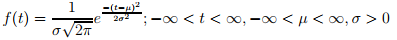(12.10)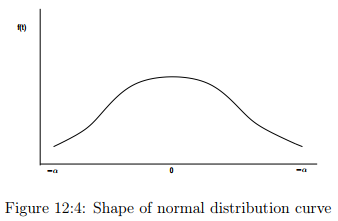where µ is the mean of the headway and σ is the standard deviation of the headways. The shape of the probability density function is shown in figure 12:4. The probability that the time headway (t) less than a given time headway (h) is given by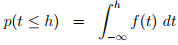(12.11)

and the value of this is shown as the area under the curve in figure 12:5 (a) and the probability of time headway (t) less than a given time headway (h + δh) is given by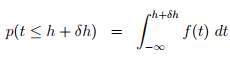(12.12)

This is shown as the area under the curve in figure 12:5 (b). Hence, the probability that the time headway lies in an interval, say h and h + δh is given by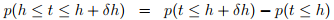(12.13)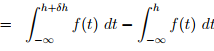This is illustrated as the area under the curve in figure 12:5 (c). Although the probability for headway for an interval can be computed easily using equation 12.13, there is no closed form solution to the equation 12.11. Eventhough it is possible to solve the above equation by numerical integration, the computations are time consuming for regular applications. One way to overcome this difficulty is to use the standard normal distribution table which gives the solution to the equation 12.11 for a standard normal distribution. A standard normal distribution is normal distribution of a random variable whose mean is zero and standard deviation is one. The probability for any random variable, having a mean (µ) and standard deviation (σ) can be computed by normalizing that random variable with respect to its mean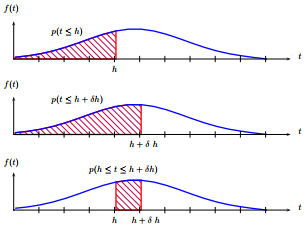Figure 12:5: Illustration of the expression for probability that the random variable lies in an interval for normal distribution and standard deviation and then use the standard normal distribution table. This is based on the concept of normalizing any normal distribution based on the assumption that if t follows normal distribution with mean µ and standard deviation σ, then (t − µ)/σ follows a standard normal distribution having zero mean and unit standard deviation. The normalization steps shown below.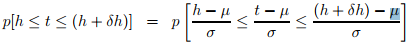(12.14)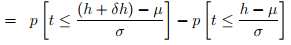The first and second term in this equation be obtained from standard normal distribution table. The following example illustrates this procedure.Numerical Example

If the mean and standard deviation of certain observed set of headways is 2.25 and 0.875 respectively, then compute the probability that the headway lies in an interval of 1.5 to 2.0 seconds.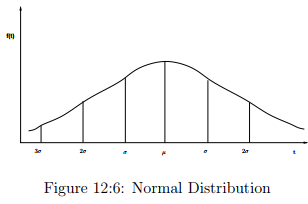Solution: The probability that headway lies between 1.5 and 2.0 can be obtained using equation 12.14, given that µ = 2.25 and σ = 0.85 as: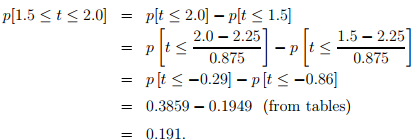Note that the p(t ≤ −0.29) and p(t ≤ −0.80) are obtained from the standard normal distribution tables. Since the normal distribution is defined from −α to +α unlike an exponential distribution which is defined only for positive number, it is possible that normal distribution may generate negative headways. A practical way of avoiding this is to shift the distribution by some value so that it will mostly generate realistic headways. The concept is illustrated in figure 12:6. Suppose α is the minimum possible headway and if we set α = µ − σ than about 60% of headway will be greater than α. Alternatively, if we set α = µ − 2σ, than about 90% of the headway will be greater than α. Further, if we set α = µ − 3σ, than about 99% of the headway will be greater than α. To generalize,

α = µ − nσ                                           (12.15)

Numerical Example

Given that observed mean headway is 3.5 seconds and standard distribution is 2.6 seconds, then compute the probability that the headway lies between 0 and 0.5. Assume that the minimum expected headway is 0.5 seconds.Solution: First, compute the standard deviation to be used in calculation using equation 12.15, given that µ = 3.5, σ = 2.6,

and α = 0.5. Then: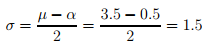(12.15)

Second, compute the probability that headway less than zero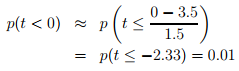The value 0.01 is obtained from standard normal distribution table. Similarly, compute the probability that headway less than 0.5 as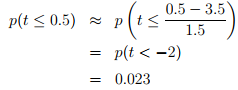The value 0.23 is obtained from the standard normal distribution table. Hence, the probability that headway lies between 0 and 0.5 is obtained using equation 12.14 as p(0 ≤ t ≤ 0.5)=0.023− 0.010 = 0.023.

Numerical Example

An observation from 2434 samples is given table below. Mean headway observed was 3.5 seconds and the standard deviation observed was 2.6 seconds. Fit a normal distribution, if we assume minimum expected headway is 0.5.

Solutions

The given headway range and the observed probability is given in column (2), (3) and (4). The observed frequency for the first interval (0 to 1) can be computed as the product of observed frequency pi and the number of observation (N) i.e.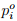= pi×N = 0.012×2434 = 29.21 as shown in column (5). Compute the standard deviation to be used in calculation, given that µ = 3.5, σ = 2.6, and α = 0.5 as: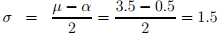h h + dh P°i 0.0 1.0 0.012 1.0 2.0 0.178 2.0 3.0 0.316 3.0 4.0 0.218 4.0 5.0 0.108 5.0 6.0 0.055 6.0 7.0 0.033 7.0 8.0 0.022 8.0 9.0 0.013 0.0 > 0.045 Total 1.00

Second, compute the probability that headway less than zero.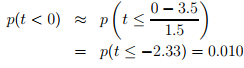The value 0.01 is obtained for standard normal distribution table is shown in column (6). Similarly, compute the probability that headway less than 1.0 as: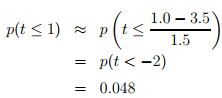The value 0.048 is obtained from the standard normal distribution table is shown in column (6). Hence, the probability that headway between 0 and 1 is obtained using equation 12.14 as p(0 ≤ t ≤ 1)=0.048 − 0.010 = 0.038 and is shown in column (7). Now the computed frequency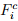is p(t < h < t + 1) ×N = 0.038 ×2434 = 92.431 and is given in column (8). This procedure is repeated for all the subsequent items. It may be noted that probability of headway > 9.0 is computed by one minus probability of headway less than 9.0 = 1−(0.038+0.111+. . .) = 0.010.

Table 12:4: Solution using normal distribution

 No(1) h(2) h + δ h(3)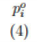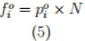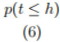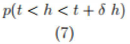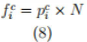1 0.0 1.0 0.012 29.21 0.010 0.038 92.431 2 1.0 2.0 0.178 433.25 0.048 0.111 269.845 3 2.0 3.0 0.316 769.14 0.159 0.211 513.053 4 3.0 4.0 0.218 530.61 0.369 0.261 635.560 5 4.0 5.0 0.108 262.87 0.631 0.211 513.053 6 5.0 6.0 0.055 133.87 0.841 0.111 269.845 7 6.0 7.0 0.033 80.32 0.952 0.038 92.431 8 7.0 8.0 0.022 53.55 0.990 0.008 20.605 9 8.0 9.0 0.013 31.64 0.999 0.001 2.987 10 9.0 > 0.045 109.53 1.000 0.010 24.190 Total 2434

Pearson Type III distribution

As noted earlier, the intermediate flow is more complex since certain vehicles will have interaction with the other vehicles and certain may not. Here, Pearson Type III distribution can be used for modelling intermediate flow. The probability density function for the Pearson Type III distribution is given as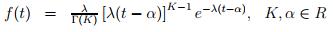(12.17)

where λ is a parameter which is a function of µ, K and α, and determine the shape of the distribution. The term µ is the mean of the observed headways, K is a user specified parameter greater than 0 and is called as a shift parameter. The Γ() is the gamma function and given as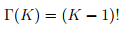(12.18)

It may also be noted that Pearson Type III is a general case of Gamma, Erlang and Negative Exponential distribution as shown in below: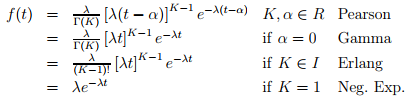The expression for the probability that the random headway (t) is greater than a given headway (h), p(t ≥ h), is given as: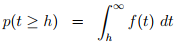(12.19)

and similarly p(t > h + δh) is given as: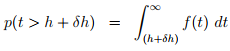(12.20)

and hence, the probability that the headway between h and h + δh is given as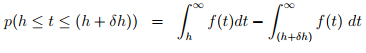(12.21)

It may be noted that closed form solution to equation 12.19 and equation 12.20 is not available. Numerical integration is also difficult due to computational requirement. Using table as in the case of Normal Distribution is difficult, since the table will be different for each K. A common way of solving this is by using the numerical approximation to equation 12.21. The solution to equation 12.21 is essentially the area under the curve defined by the probability density function between h and h + δh. If we assume that line joining f(h) and f(h + δh) is linear, which is a reasonable assumption if δh is small, than the are under the curve can be found out by the following approximate expression: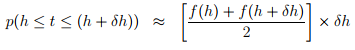(12.22)

This concept is illustrated in figure 12:7

Stepwise procedure to fit a Pearson Type III distribution
1. Input required: the mean (µ) and the standard deviation (σ) of the headways.
2. Set the minimum expected headway (α). Say, for example, 0.5. It means that the p(t < 0.5) ≈ 0.
3. Compute the shape factor using the mean (µ) the standard deviation (σ) and the minimum expected headway (α)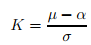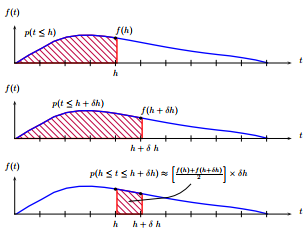Figure 12:7: Illustration of the expression for probability that the random variable lies in an interval for Person Type III distribution

4. Compute the term flow rate (λ) as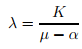Note that if K = 1 and α = 0, then λ = 1/μ which is the flow rate.

5. Compute gamma function value for K as: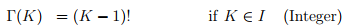(12.23)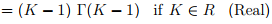Although the closed form solution of Γ(K) is available, it is difficult to compute. Hence, it can be obtained from gamma table. For, example: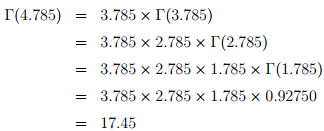Note that the value of Γ(1.785) is obtained from gamma table for Γ(x) which is given for 1 ≤ x ≤ 2.

6. Using equation 12.17 solve for f(h) by setting t = h where h is the lower value of the range and f(h+δh) by setting t = h+δh where (h+δh) is the upper value of the headway range. Compute the probability that headway lies between the interval of h and h + δh using equation 12.22.

The Gamma function can be evaluated by the following approximate expression also: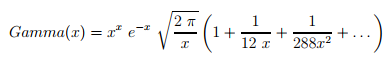(12.24)

Numerical Example

An observation from 2434 samples is given table below. Mean headway observed was 3.5 seconds and the standard deviation 2.6 seconds. Fit a Person Type III Distribution.

 h h + dh P°i 0.0 1.0 0.012 1.0 2.0 0.178 2.0 3.0 0.316 3.0 4.0 0.218 4.0 5.0 0.108 5.0 6.0 0.055 0.0 7.0 0.033 7.0 8.0 0.022 8.0 9.0 0.013 9.0 > 0.045 Total 1.00

Solutions Given that mean headway (µ) is 3.5 and the standard deviation (σ) is 2.6. Assuming the expected minimum headway (α) is 0.5, K can be computed as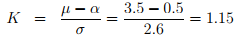and flow rate term λ as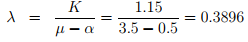Now, since K = 1.15 which is between 1 and 2, Γ(K) can be obtained directly from the gamma table as Γ(K) = 0.93304. Here, the probability density function for this example can be expressed as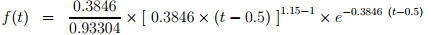The given headway range and the observed probability is given in column (2), (3) and (4). The observed frequency (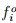) for the first interval (0 to 1) can be computed as the product of observed proportion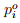and the number of observations (N). That is,=i ×N = 0.012×2434 = 29.21 as shown in column (5). The probability density function value for the lower limit of the first interval (h=0) is shown in column (6) and computed as: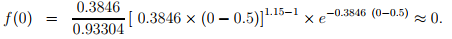Note that since t−α (0−0.5) is negative and K −1 (1.15−1) is a fraction, the above expression cannot be evaluated and hence approximated to zero (corresponding to t=0.5). Similarly, the probability density function value for the lower limit of the second interval (h=1) is shown in column 6 and computed as: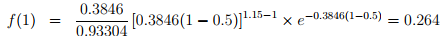Now, for the first interval, the probability for headway between 0 and 1 is computed by equation ?? as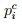(0 ≤ t ≤ 1) =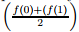× (1 − 0) = (0 + 0.0264)/2 × 1 = 0.132 and is given in column (7). Now the computed frequency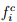is× N = 0.132 × 2434 = 321.1 and is given in column (8). This procedure is repeated for all the subsequent items. It may be noted that probability of headway > 9 is computed by 1-probability of headway less than 9 = 1 − (0.132 + 0.238 + . . .) = 0.044. The comparison of the three distribution for the above data is plotted in Figure 12:8.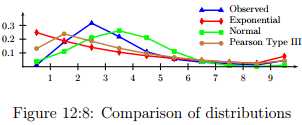Offer running on EduRev: Apply code STAYHOME200 to get INR 200 off on our premium plan EduRev Infinity!

## Transportation Engineering

23 videos|66 docs|39 tests

,

,

,

,

,

,

,

,

,

,

,

,

,

,

,

,

,

,

,

,

,

;